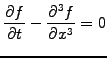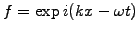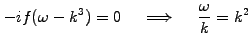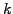SYLLABUS  Previous: 1.3.2 Diffusion  Up: 1.3 Prototype problems  Next: 1.3.4 Wave-breaking

### 1.3.3 Dispersion

Slide : [ dispersion || VIDEO login]

Dispersion occurs when different wavelengths propagate with different phase velocities. Take for example a third order dispersion equation(1.3.3#eq.1)

The harmonic ansatz in space and timeshows that the phase velocity(1.3.3#eq.2)

is larger for short wavelengths (largepropagate faster) than long wavelengths (smallpropagate slower). In the Korteweg-DeVries equation, this will explain why large amplitude solitons with short wavelengths propagate more rapidly than low amplitudes solitons having long wavelengths.

Unfortunately, dispersion does not always have a physical origin: remember (1.2.4#eq.3), showing how a centered finite difference approximation under-estimates first order derivatives of short wavelengths. This is exactly what happens in the 3 levels scheme for the advection equation, where different Fourier components included in the initial square box function propagate with different velocities.

 Numerical experiments: dispersion Switch from a Box to a Gaussian initial condition to make sure that long wavelengths do indeed propagate with the right phase velocity. Pushing the numerical approximation to the extreme, now switch to a Cosine with less than two mesh points per wavelength ICWavelenth=1.9375. Observe how the short wavelength oscillations propagate backward, i.e. in the opposite direction from what they are expected in presence of advection only. Discuss what happens if a harmonic function is initialized with exactly 2 mesh points per wavelength.

Numerical dispersion affects almost every approximation that will be discussed in this course. It is therefore important to ensure that numerical solutions reproduce the physical dispersion and not the spurious numerical dispersion introduced by the discretisation.

SYLLABUS  Previous: 1.3.2 Diffusion  Up: 1.3 Prototype problems  Next: 1.3.4 Wave-breaking